Skip to main content

Thank you for visiting nature.com. You are using a browser version with limited support for CSS. To obtain the best experience, we recommend you use a more up to date browser (or turn off compatibility mode in Internet Explorer). In the meantime, to ensure continued support, we are displaying the site without styles and JavaScript.

# A universal test for gravitational decoherence

## Abstract

Quantum mechanics and the theory of gravity are presently not compatible. A particular question is whether gravity causes decoherence. Several models for gravitational decoherence have been proposed, not all of which can be described quantum mechanically. Since quantum mechanics may need to be modified, one may question the use of quantum mechanics as a calculational tool to draw conclusions from the data of experiments concerning gravity. Here we propose a general method to estimate gravitational decoherence in an experiment that allows us to draw conclusions in any physical theory where the no-signalling principle holds, even if quantum mechanics needs to be modified. As an example, we propose a concrete experiment using optomechanics. Our work raises the interesting question whether other properties of nature could similarly be established from experimental observations alone—that is, without already having a rather well-formed theory of nature to make sense of experimental data.

## Introduction

Experiments1,2,3,4 aiming at testing the presence—and amount—of gravitational decoherence generally go beyond established theory. Many theoretical models for gravitational decoherence have been proposed5,6,7,8,9,10,11,12,13,14,15,16,17,18,19,20,21,22,23,24,25, and it is wide open if one of these proposals is correct. As such, experiments are of a highly exploratory nature, aiming to establish data points that constrain rival theoretical proposals. This task is made even more difficult by the fact that quantum mechanics and gravity do not go hand in hand, and indeed quantum mechanics may need to be modified in a yet unknown way in order to account for gravitational effects such as decoherence. We are thus compelled to design an experiment that provides a guiding light for the search for the right theoretical model—or indeed new physical theory—whose conclusions do not rely on quantum mechanics.

Here we propose an experimental procedure to estimate gravitational decoherence whose conclusions hold even if quantum mechanics would need to be modified. We first establish a general information-theoretic notion of decoherence which reduces to the standard measure within quantum mechanics. Second, drawing on ideas from quantum information, we propose a very general experiment that allows us to obtain a quantitative estimate of decoherence of any physical process for any physical theory satisfying only very mild conditions. Our method is fully general and could in principle be used to supplement many existing experimental proposals in a way that would allow us to draw conclusions from data even if quantum mechanics would need to be modified. Concretely, if a process (supposedly) causing gravitational decoherence can be probed experimentally, then our general method allows us to measure a parameter β that translates into an upper bound on decoherence,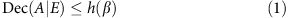where Dec(A|E) is the amount of decoherence of a system A with respect to its environment E (we will define this below). The function h is plotted in the Discussion section for quantum mechanics, but also very general physical theories. As an example, we propose a concrete experiment using optomechanics to estimate gravitational decoherence in any such theory, including quantum mechanics as a special case. We note that our procedure could be used to probe any form of decoherence, but only in the case gravitational decoherence is there a pressing motivation for considering theories beyond quantum mechanics.

## Results

### Decoherence in quantum mechanics

Before we turn to our general approach (see Fig. 1), let us first focus on the concept of decoherence within quantum mechanics as an easy warm-up. This demonstrates some principles that we will generalize to a broad framework of theories in the following section. Here we first show how the protocol given in Fig. 2 allows us to estimate quantum mechanical decoherence without knowing the decoherence process, and without doing quantum tomography to determine it. Traditionally, the presence of decoherence within quantum mechanics is related to the change of state due to measurement and the ‘collapse of the wavefunction’. Decoherence is thereby often seen as a decay of the off-diagonal terms in the density operator ρ, corresponding to a (weak) measurement of the state. It is clear that this way of thinking about decoherence is entirely tied to the quantum mechanical matrix formalism, and also offers little in the way of quantifying the amount of decoherence in an operationally meaningful way.Figure 1: Illustration of our approach.Figure 2: Diagram of the general setup.

The modern way of understanding decoherence in quantum mechanics in a quantitative way is provided by quantum information theory. One thereby thinks of a decoherence process as an interaction of a system A′ with an environment as described in Fig. 2, resulting in a quantum channel ΓA′→B. The amount of decoherence can now be quantified by the channel’s ability to transmit quantum information, that is, its quantum capacity (see Supplementary Note 1 for further background). For a finite number of channels, the relevant quantity is the single-shot capacity as determined by the so-called min-entropy Hmin(A|E)26,27.

Apart from its information-theoretic significance, the min-entropy has a beautiful operational interpretation that also makes its role as a decoherence measure intuitively apparent. Very roughly, the amount of decoherence can be understood as a measure of how correlated E becomes with A. Suppose we start with a maximally entangled test state ΦAA where the decoherence process is applied to A′. This results in a state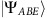(see Fig. 2). If no decoherence occurs, the output state will be of the form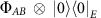where A′=B. That is, A and B are maximally entangled, but A and E are completely uncorrelated. The strongest decoherence, however, produces an output state of the form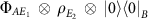where A′=E1 and where E is subdivided into subsystems E=E1E2. That is, A is now maximally entangled with E1, whereas A and B are completely uncorrelated.

What about the intermediary regime? The min-entropy can be written as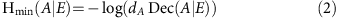where dA is the dimension of A, and (ref. 28)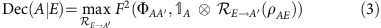and where F denotes the fidelity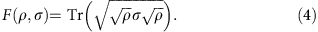The maximization above is taken over all quantum operations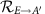on the system E, which aim to bring the state ρAE as close as possible to the maximally entangled state ΦAA (see Fig. 3). Intuitively, Dec(A|E) can thus be understood as a measure of how far the output ρAE is from the setting of maximum decoherence (where ρAEAE is the maximally entangled state). If there is no decoherence, we have ρAE=/dAρE giving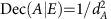and Hmin(A|E)=log dA. If there is maximum decoherence, we have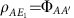giving Dec(A|E)=1 and Hmin(A|E)=−log dA where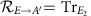is simply the operation that discards the remainder of the environment E2. A larger value of Dec(A|E) thus corresponds to a larger amount of decoherence. In the quantum case, Dec(A|E) can be computed using any semi-definite programming solver29,30. We remark that Dec(A|E) does itself not depend on the dimension of the system A. Furthermore, we note that Dec(A|E) does not depend on the particular physical realization of the system A, but merely the amount of information that it can hold. We point out that this entanglement-preservation picture is equivalent to the picture in which the quantum state of a single system decoheres31 (see Fig. 4).Figure 3: Intuitive picture of the decoherence quantity.Figure 4: Equivalence of entanglement preservation and single system decoherence.

We hence see that in quantum mechanics, the relevant measure of decoherence is simply Dec(A|E) (see Fig. 5 for some examples). How can we estimate it in an experiment? Our goal in deriving this estimate will be to rely on concepts that we can later extend beyond the realm of quantum theory, deriving a universally valid test. It is clear that to estimate Dec(A|E) we need to make a statement about the entanglement between A and E—yet E is inaccessible to our experiment. A property of quantum mechanics known as the monogamy of entanglement32 nevertheless allows such an estimate: if ρAB is highly entangled, then ρAE is necessarily far from highly entangled. Since low entanglement in ρAE means that Dec(A|E) is low, a test that is able to detect entanglement between A and B should help us bound Dec(A|E) from above.Figure 5: Comparison of the quantum bound with the actual values of Dec(A|E)ρ for some example channels and measurements.

### Beyond quantum mechanics

The real challenge is to show that the conclusions of our test remain valid even outside of quantum mechanics. Since we want to make as few assumptions as possible, we consider the most general probabilistic theory, in which we are only given a set of possible states Ω and measurements on these states. Every measurement is thereby a collection M={ea}a of effects ea:Ω→[0, 1] satisfying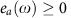and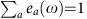for all ωΩ. The label a corresponds to a measurement outcome ‘a’. The notion of separated systems A, B and E is in general difficult to define uniquely. We thus again make the most minimal assumption possible in which we identify ‘systems’ A, B and E with sets of measurements that can be performed. In a nutshell, we make the following assumptions: there is a notion of states and measurements, we can observe measurement outcomes that occur with some probability, we identify subsystems by sets of possible measurements, and the no-signalling principle holds (see Supplementary Notes 3 and 4 for details).

The first obstacle consists of defining a general notion of decoherence. We saw that quantumly decoherence can be quantified by how well correlations between A and A′ are preserved, and this can be measured by how well the decoherence process preserves the maximally correlated (that is, entangled) state. Indeed, we can also quantify classical noise in terms of how well it preserves correlations, where the maximally correlated state takes on the form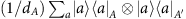for some classical symbols a. We hence start by defining the set of maximally correlated states, by observing a crucial and indeed defining property of the maximally correlated state in quantum mechanics. Concretely, A and A′ are maximally entangled if and only if for any von Neumann measurement on A, there exists a corresponding measurement on A′ giving the same outcome. Again, the same is also true classically but made trivial by the fact that there is only one measurement. In analogy, we thus define the set of maximally correlated states as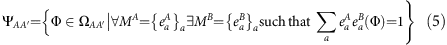This set coincides with the set of maximally entangled states in quantum mechanics, where A′ can potentially contain an additional component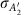in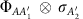which is irrelevant to our discussion. We thus define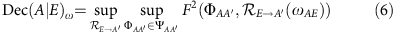where ωAE is the state shared between A and E according to the general physical theory. The fidelity between two states ω1 and ω2 is thereby defined in full analogy to the quantum case33 as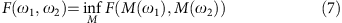where the minimization is taken over all possible measurements M, and M(ω) denotes the probability distribution over the measurement outcomes of M. Here, the fidelity F(M(ω1), M(ω2)) can be written as33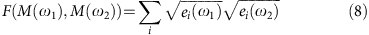where the sum ranges over all effects ei of the measurement M (see Supplementary Note 3 for further details). That is, the fidelity can be expressed as the minimum fidelity between probability distributions of classical measurement outcomes. We will not need to make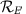explicit in order to bound Dec(A|E). Equation (6) gives us the familiar quantity within quantum mechanics, but provides us with a very intuitive way to quantify decoherence in any physical theory that admits maximally correlated states. We emphasize that with our general techniques the latter demand could be weakened to allow all theories, even those which only have (weak) approximations of maximally correlated states.

The second challenge is to prove that our test actually provides a bound on Dec(A|E)ω. Note that without quantum mechanics to guide us, all that we could reasonably establish by performing measurements on A and B are the probabilities of outcomes a and b given measurement settings x and y. That is, the probability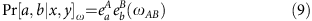where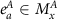and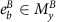. Yet, given the system E is entirely inaccessible to us we have no hope of measuring Pr[a, b, c|x, y, z]ω directly, where z denotes a measurement setting on E with outcome c. Nevertheless, similar to quantum entanglement, it is known that non-signalling distributions are again monogamous34—and it is this fact that allows us to draw conclusions about E by measuring only A and B. We will therefore make a non-trivial assumption about the physical theory, namely that no-signalling holds between A, B and E. We emphasize that weaker constraints on the amount of signalling could also lead to a bound—but we are not aware of any other concrete example to consider. Mathematically, no-signalling means that the marginal distributions obey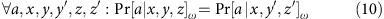that is, the choice of measurement settings y, y′ and z, z′ does not influence the probability distribution over the outcomes a. A set of distributions is non-signalling if such conditions hold for all marginal distributions.

## Discussion

What have we actually learned when performing such an experiment? We first observe that the measured β always gives an upper bound on the amount of decoherence observed—for any non-signalling theory. This means that even if quantum mechanics would indeed need to be modified we can still draw conclusions from the data we obtain. As such, the observations made in such an experiment establish a fundamental limit on decoherence no matter what the theory might actually look like in detail. It is clear, however, that the bound thus obtained is much weaker than if we had assumed quantum mechanics. No-signalling is but one of many principles obeyed by quantum mechanics, and these other features put stronger bounds on the values that Dec(A|E) can take. Our motivation for considering theories which are only constrained by no-signalling is to demonstrate even such weak demands still allow us to draw meaningful conclusions from such an experiment. One can easily adapt our approach by introducing further constraints on the probabilities Pr[a, b, c|x, y, z]—but not all of quantum mechanics—in order to get stronger bounds. Also in a fully quantum mechanical world, our approach yields a bound (see Fig. 6). If we assume quantum mechanics, we may of course also try and perform process tomography in order to determine the decoherence process, and indeed any experiment should try and perform such a tomographic analysis whenever possible. The appeal of our approach is rather that we can draw conclusions from the experimental data while making only very minimal assumptions about the underlying physical theory.Figure 6: Allowed values of the decoherence quantity for measured CHSH values.

One may wonder why we only upper bound Dec(A|E). Note that from our experimental statistics we can only make statements about the overall decoherence observed in the experiment, namely the gravitational decoherence (if it exists) as well as any other decoherence introduced due to experimental imperfections. Finding that the Bell violation is low (and thus maybe Dec(A|E) might be large) can thus not be attributed conclusively to the gravitational decoherence process, making a lower bound on Dec(A|E) meaningless if our desire is to make statements about a particular decoherence process such as gravity.

Second, we observe that our approach can rule out models of gravitational decoherence but not verify a particular one. It is important to note that a model for gravitational decoherence does not stand on its own, but is always part of a theory on what states, evolutions and measurements behave like. Given such a physical theory and a model for gravitational decoherence, we know enough to compute Dec(A|E), such as for example in equations (15, 16, 17). In addition, we can compute an upper bound ftheory(β) on Dec(A|E) specific to that theory, which may give a much stronger bound than no-signalling alone. Indeed, we see from Fig. 6 that this is the case for quantum mechanics. Given the calculated Dec(A|E) and the experimentally observed value for ftheory(β), we can then compare: If Dec(A|E)>ftheory(β), then the model (or indeed theory) we assumed must be wrong. However, if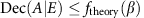, then we know that the model and theory would be consistent without experimental observations.

Note that while our framework allows for theories with super-quantum correlations (that is, with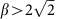(ref. 35)), it is also perfectly valid in the regime where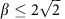. The bound shown in Fig. 6 is non-trivial for all β>2, and therefore conclusions can be drawn for all such β. Hence, the various arguments brought forward in the literature for why super-quantum correlations should not be observed36,37,38,39,40,41,42 do not contradict our work. The numeric value of the red bound in Fig. 6 may seem weak. However, recall from above that this is a bound for the most general class of theories that can be considered in our framework, while additional assumptions about the theory in question increase the strength of the bound.

Our approach thus provides a guiding light in the search for gravitational decoherence models. It is very general, and could in principle be used in conjunction with other proposed experimental setups and decoherence models. In particular, it could also be used to probe decoherence models conjectured to arise from decoherence affecting macroscopic objects, where there exist proposals to bring such objects into superposition3. Clearly, however, probing such models using entanglement is extremely challenging.

It is a very interesting open question to improve our analysis and to apply it to other physical theories that are more constrained than by no-signalling, but yet do not quite yield quantum mechanics. Candidates for this may come from the study of generalized probabilistic theories where the authors (e.g., refs 43, 44, 45, 46, 47, 48) introduced further constraints in order to recover quantum mechanics, but also from suggested ways to modify the Schrödinger equation in order to account for non-quantum mechanical noise. Since our approach could also be applied to higher dimensional systems, and other Bell inequalities, it is a very interesting open question whether other Bell inequalities could be used to obtain stronger bounds on Dec(A|E) from the resulting experimental observations.

## Methods

### In quantum mechanics

Figure 2 illustrates the general experimental procedure. As an easy warm-up, let us first again consider what happens in quantum mechanics. For now, we assume that the measurement devices have no memory. That is, the experiment behaves the same in each round, independent on the previous measurements. It is relatively straightforward to obtain an upper bound on Dec(A|E) by extending techniques from quantum key distribution49. In essence, we maximize Dec(A|E) over all states that are consistent with the observed CHSH correlator β (see Fig. 2). This maximization problem is simplified by the inherent symmetries of the CHSH inequality, allowing us to reduce this optimization problem to consider only states that are diagonal in the Bell basis. We proceed to establish properties of min and max entropies for Bell diagonal states, leading to an upper bound. Concretely, we show in Supplementary Note 2 that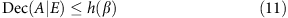where h(β) is an easy optimization problem that can be solved using Lagrange multipliers. We have chosen not to weaken this bound by an analytical bound that is strictly larger, as it is indeed easily evaluated (see Fig. 6). If the devices are allowed memory, then a variant of this test and some more sophisticated techniques from quantum key distribution can nevertheless be shown to give a bound.

### Beyond quantum mechanics

Let us first give a very loose intuition why performing a Bell experiment on A and B may allow us to bound Dec(A|E)ω. It is well known34 that non-signalling correlations are also monogamous. That is, if we observe a violation of the CHSH inequality as captured by the measured parameter β, then we know that the violation between A and E and also between E and B must be low. Note that the expectation values Tr[ρAB(AxBy)] in terms of quantum observables Ax and By can be expressed in terms of probabilities as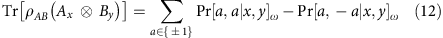where we have again used ωAB in place of ρAB to remind ourselves that we may be outside of QM. Let us now assume by contradiction that the state ωAE shared between A and E would be close to maximally correlated. Then by definition of the maximally correlated state, for every measurement on A, there exists some measurement on E which yields the same outcome with high probability. Hence, if ωAE would be close to maximally correlated, then we would expect that E and B can achieve a similar CHSH violation as A and B—because E can make measurements that reproduce the same correlations that A can achieve with B. Yet, we know that this cannot be since CHSH correlations are monogamous. Note that a map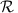(as in Fig. 3), followed by a measurement in fact constitutes another measurement. Hence, considering all possible measurements that Eve can perform, we cover all such possible mapsthat Eve might want to apply.

While we do not follow the exact steps suggested by this intuition, we employ a technique in Supplementary Note 3 that has also been used for studying monogamy of CHSH correlations34. Specifically, we use linear programming as a technique to obtain bounds. We thereby first relate the fidelity to the statistical distance, which is a linear functional. We are then able to optimize this linear functional over probability distributions Pr[a, b, c|x, y, z]ω satisfying linear constraints. The first such constraint is given by the fact that we consider only non-signalling distributions. The second is the fact that the marginal distribution Pr[a, b|x, y]ω leads to the observed Bell violation β. The last one stems from the fact that maximal correlations can also be expressed using a linear constraint. Solving this linear program for an observed violation β leads to Fig. 6.

### Optomechanical experiment

To gain insights into the significance of gravitational decoherence, we examine Diosi’s theory of gravitational decoherence6 as an example. This is equivalent to the decoherence model introduced in Kafri et al.10. We show in Supplementary Note 6 how Dec(A|E) can be evaluated for many other decoherence processes, opening the door for applying our method to many other possible experiments. Diosi’s model can be applied to an optomechanical cavity in which one mirror is free to move in a harmonic potential with frequency ωm as in Fig. 7. The master equation for a massive particle moving in a harmonic potential, including gravitational decoherence isFigure 7: Probing an optomechanical system.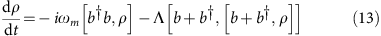where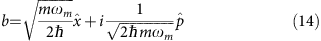with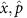the usual canonical position and momentum operators for the moving mirror. We have that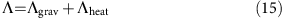where the gravitational decoherence rate Λgrav is given by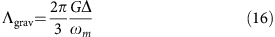with G the Newton gravitational constant and Δ the density of the moving mirror. As one might expect Λgrav is quite small, of the order of 10−8 s−1 for suspended mirrors with ωm1. The term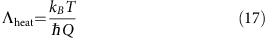with Q=ω/γm corresponds to mechanical heating. To see the effect of the gravitational term stand out next to the mechanical heating we thus need to make the temperature T low. A calculation shows that this model leads to a dephasing channel Γ(ρ)=+(1−p)ZρZ where p is a function of the density Δ, and the other parameters. In Supplementary Note 6, we show that for this model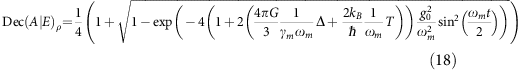where G is the Newton gravitational constant, kB is the Boltzmann constant, and ħ the Planck constant (see Fig. 8 for the other parameters).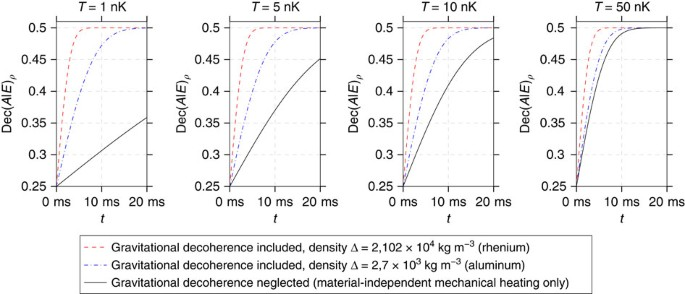Figure 8: Predicted values of the decoherence quantity in the optomechanical experiment.

### Code availability

The source code of the semidefinite program and the linear program used to derive the plots in Fig. 6 are available from the authors on request.

### Data availability

Data sharing not applicable to this article as no data sets were generated or analysed during the current study.

## Additional information

How to cite this article: Pfister C. et al. A universal test for gravitational decoherence. Nat. Commun. 7, 13022 doi: 10.1038/ncomms13022 (2016).

## References

1. 1

Pepper, B., Ghobadi, R., Jeffrey, E., Simon, C. & Bouwmeester, D. Optomechanical superpositions via nested interferometry. New J. Phys. 14, 115025 (2012).

2. 2

Marshall, W., Simon, C., Penrose, R. & Bouwmeester, D. Towards quantum superpositions of a mirror. Phys. Rev. Lett. 91, 130401 (2003).

3. 3

Romero-Isart, O. et al. Large quantum superpositions and interference of massive nanometer-sized objects. Phys. Rev. Lett. 107, 020405 (2011).

4. 4

Pikovski, I., Vanner, M. R., Aspelmeyer, M., Kim, M. & Brukner, C. Probing planck-scale physics with quantum optics. Nat. Phys. 8, 393–397 (2012).

5. 5

Penrose, R. On gravity’s role in quantum state reduction. Gen. Relat. Gravit. 28, 581 (1996).

6. 6

Diósi, L. Models for universal reduction of macroscopic quantum fluctuations. Phys. Rev. A 40, 1165–1174 (1989).

7. 7

Diosi, L. The gravity-related decoherence master equation from hybrid dynamics. J. Phys.: Conf. Ser. 306, 012006 (2011).

8. 8

Diosi, L. Gravitation and quantummechanical localization of macroobjects. Phys. Lett. A 105, 199 (1984).

9. 9

Diosi, L. A universal master equation for the gravitational violation of quantum mechanics. Phys. Lett. 120, 377–381 (1987).

10. 10

Kafri, D., Taylor, J. M. & Milburn, G. J. A classical channel model for gravitational decoherence. New J. Phys. 16, 065020 (2014).

11. 11

Stamp, P. C. E. Environmental decoherence versus intrinsic decoherence. Phil. Trans. Roy. Soc. A 370, 4429 (2012).

12. 12

Anastopoulous, C. & Hu, B. L. A master equation for gravitational decoherence: probing the textures of spacetime. Class. Quant. Grav. 30, 165007 (2013).

13. 13

Hu, B. L. Gravitational decoherence, alternative theories, and semiclassical gravity. J. Phys.: Conf. Ser. 504, 012021 (2014).

14. 14

Anastopoulous, C. & Hu, B. L. Decoherence in quantum gravity: issues and critiques. Journal of Physics. J. Phys.: Conf. Ser. 67, 012012 (2007).

15. 15

Kay, B. Decoherence of macroscopic closed systems within newtonian quantum gravity. Class. Quant. Grav. 15, L89 (1998).

16. 16

Breuer, H. P., Göklü, E. & Lämmerzah, C. Metric fluctuations and decoherence. Class. Quant. Grav. 26, 105012 (2007).

17. 17

Wang, C., Bingham, R. & Mendoca, J. T. Quantum gravitational decoherence of matter waves. Class. Quant. Grav. 23, L59 (2006).

18. 18

Ghirardi, G. C., Rimini, A. & Weber, T. Unified dynamics for microscopic and macroscopic systems. Phys. Rev. D 34, 470–491 (1986).

19. 19

Pearle, P. Combining stochastic dynamical state-vector reduction with spontaneous localization. Phys. Rev. A 39, 2277–2289 (1989).

20. 20

Ghirardi, G. C., Pearle, P. & Rimini, A. Markov processes in hilbert space and continuous spontaneous localization of systems of identical particles. Phys. Rev. A 42, 78–89 (1990).

21. 21

Pearle, P. Ways to describe dynamical state-vector reduction. Phys. Rev. A 48, 913–923 (1993).

22. 22

Pearle, P. Completely quantized collapse and consequences. Phys. Rev. A 72, 022112 (2005).

23. 23

Pearle, P. How stands collapse i. J. Phys. A 40, 3189 (2007).

24. 24

Pearle, P. Stress tensor for quantized random field and wave-function collapse. Phys. Rev. A 78, 022107 (2008).

25. 25

Pikovski, I., Zych, M., Costa, F. & Brukner, C. Stress tensor for quantized random field and wave-function collapse. Nat. Phys. 11, 668–672 (2008).

26. 26

Dupuis, F., Berta, M., Wullschleger, J. & Renner, R. One-shot decoupling. Commun. Math. Phys. 328, 251–284 (2014).

27. 27

Buscemi, F. & Datta, N. The quantum capacity of channels with arbitrarily correlated noise. IEEE Trans. Inform. Theory 56, 1447–1460 (2010).

28. 28

König, R., Renner, R. & Schaffner, C. The operational meaning of min- and max-entropy. IEEE Trans. Inf. Theory 55, 4337–4347 (2009).

29. 29

Renner, R. Security of Quantum Key Distribution PhD thesis (ETH Zürich (2005).

30. 30

Sturm, J. Using SeDuMi 1.02, a MATLAB toolbox for optimization over symmetric cones. Optim. Method. Softw. 11-12, 625–653 (1999).

31. 31

Barnum, H., Knill, E. & Nielsen, M. A. On quantum fidelities and channel capacities. IEEE Trans. Inform. Theory 46, 1317–1329 (2000).

32. 32

Terhal, B. Is entanglement monogamous? IBM J. Res. Dev. 48, 71–78 (2004).

33. 33

Nielsen, M. A. & Chuang, I. L. Quantum Computation and Quantum Information Cambridge University Press (2000).

34. 34

Toner, B. Monogamy of non-local quantum correlations. Proc. R. Soc. A 465, 59–69 (2009).

35. 35

Tsirelson, B. S. Quantum generalizations of bell’s inequality. Lett. Math. Phys. 4, 93–100 (1980).

36. 36

Rohrlich, D. Stronger-than-quantum bipartite correlations violate relativistic causality in the classical limit. Preprint at http://arxiv.org/abs/1408.3125 (2014).

37. 37

Pawlowski, M. et al. Information causality as a physical principle. Nature 461, 1101–1104 (2009).

38. 38

Oppenheim, J. & Wehner, S. The uncertainty principle determines the nonlocality of quantum mechanics. Science 330, 1072–1074 (2010).

39. 39

Popescu, S. Nonlocality beyond quantum mechanics. Nat. Phys. 10, 264–270 (2014).

40. 40

Brunner, N., Cavalcanti, D., Pironio, S., Scarani, V. & Wehner, S. Bell nonlocality. Rev. Mod. Phys. 86, 419–478 (2014).

41. 41

van Dam, W. Implausible consequences of superstrong nonlocality. Natur. Comput. 12, 9–12 (2013).

42. 42

Dahlsten, O. C. O., Lercher, D. & Renner, R. Tsirelson’s bound from a generalized data processing inequality. New J. Phys. 14, 063024 (2012).

43. 43

Masanes, L. & Müller, M. P. A derivation of quantum theory from physical requirements. New J. Phys. 13, 063001 (2011).

44. 44

Masanes, L., Müller, M. P., Augusiak, R. & Pérez-García, D. Existence of an information unit as a postulate of quantum theory. Proc. Natl Acad. Sci. USA 110, 16373–16377 (2013).

45. 45

Chiribella, G., D’Ariano, G. M. & Perinotti, P. Informational derivation of quantum theory. Phys. Rev. A 84, 012311 (2011).

46. 46

Dakic, B. & Brukner, C. in Deep Beauty: Understanding the Quantum World through Mathematical Innovation ed. Halvorson H. 365–392Cambridge University Press (2011).

47. 47

Ududec, C. Perspectives on the Formalism of Quantum Theory PhD thesis (Univ. Waterloo (2012).

48. 48

Pfister, C. & Wehner, S. An information-theoretic principle implies that any discrete physical theory is classical. Nat. Commun. 4, 1851 (2013).

49. 49

Acín, A. et al. Device-independent security of quantum cryptography against collective attacks. Phys. Rev. Lett. 98, 230501 (2007).

50. 50

Horodecki, R., Horodecki, P. & Horodecki, M. Violating Bell inequality by mixed spin-1/2 states: necessary and sufficient condition. Phys. Lett. A 200, 340–344 (1995).

51. 51

Popescu, S. & Rohrlich, D. Generic quantum nonlocality. Phys. Lett. A 166, 293–297 (1992).

52. 52

Popescu, S. & Rohrlich, D. Which states violate bell’s inequality maximally? Phys. Lett. A 169, 411–414 (1992).

53. 53

Popescu, S. Bell’s inequalities and density matrices: revealing ‘hidden’ nonlocality. Phys. Rev. Lett. 74, 2619 (1995).

54. 54

Popescu, S. Bell’s inequalities versus teleportation: what is nonlocality? Phys. Rev. Lett. 72, 797–799 (1994).

55. 55

Nisbet-Jones, B. R., Dilley, J., Ljunggren, D. & Kuhn, A. Highly efficient source for indistinguishable single photons of controlled shape. New J. Phys. 13, 103036 (2011).

56. 56

Kessler, T. et al. A sub-40-mhz-linewidth laser based on a silicon single-crystal optical cavity. Nat. Photonics 6, 687 (2012).

Download references

## Acknowledgements

We thank Markus P. Müller, Matthew Pusey, Tobias Fritz, Gary Steele, Jonas Helsen and Thinh Le Phuc for insightful discussions. C.P., J.K., M.T., A.M., R.S. and S.W. were supported by MOE Tier 3A grant ‘Randomness from quantum processes’, NRF CRP ‘Space-based QKD’. S.W. was also supported by STW, Netherlands, an NWO VIDI, and an ERC Starting Grant. N.M. and G.M. were supported by ARC Centre of Excellence for Engineered Quantum Systems, CE110001013.

## Author information

Authors

### Contributions

S.W. devised the project, the main conceptual ideas and proof outline. C.P. worked out almost all of the technical details, and performed the numerical calculations for the suggested experiment. J.K. worked out the bound for quantum mechanics, with help from M.T. and A.M. R.S. verified the numerical results of the linear program by an independent implementation. N.M. and G.M. proposed the optomechanical experiment in discussions with S.W. C.P., J.K., G.M. and S.W. wrote the manuscript.

### Corresponding author

Correspondence to S. Wehner.

## Ethics declarations

### Competing interests

The authors declare no competing financial interests.

## Supplementary information

### Supplementary Information

Supplementary Figures 1-15, Supplementary Notes 1-6 and Supplementary References. (PDF 3373 kb)

## Rights and permissions

This work is licensed under a Creative Commons Attribution 4.0 International License. The images or other third party material in this article are included in the article’s Creative Commons license, unless indicated otherwise in the credit line; if the material is not included under the Creative Commons license, users will need to obtain permission from the license holder to reproduce the material. To view a copy of this license, visit http://creativecommons.org/licenses/by/4.0/

Reprints and Permissions

## Further reading

• ### Information Scrambling versus Decoherence—Two Competing Sinks for Entropy

• Akram Touil
•  & Sebastian Deffner

PRX Quantum (2021)

• ### Polarization gradient cooling and trapping of charged and neutral microspheres

• Ziqiang He
•  & Guangjiong Dong

Journal of the Optical Society of America B (2021)

• ### Einstein-Podolsky-Rosen entanglement and asymmetric steering between distant macroscopic mechanical and magnonic systems

• Huatang Tan
•  & Jie Li

Physical Review Research (2021)

• ### Strong mechanical squeezing for a levitated particle by coherent scattering

• Ondřej Černotík
•  & Radim Filip

Physical Review Research (2020)

• ### Optimal estimation with quantum optomechanical systems in the nonlinear regime

• Fabienne Schneiter
• , Sofia Qvarfort
• , Alessio Serafini
• , André Xuereb
• , Daniel Braun
• , Dennis Rätzel
•  & David Edward Bruschi

Physical Review A (2020)

## Comments

By submitting a comment you agree to abide by our Terms and Community Guidelines. If you find something abusive or that does not comply with our terms or guidelines please flag it as inappropriate.

## Search

### Quick linksSign up for the Nature Briefing newsletter — what matters in science, free to your inbox daily.

Get the most important science stories of the day, free in your inbox. Sign up for Nature Briefing